## Syndicate

Research / Annual reports / Main research results of the Institute for Condensed Matter Physics of NAS of Ukraine in 2021

# Main research results of the Institute for Condensed Matter Physics of NAS of Ukraine in 2021The model of patchy colloids with the dipole-dipole interaction between particle centres has been studied. Previously, a similar model was applied to describe polar associative fluids, such as water and aqueous solutions. This type of model can also be used to describe a system of colloids with inhomogeneous charge distribution, e.g. solutions of globular proteins. We propose the corresponding multi-density Ornstein-Zernike equations combined with the closures in the associative mean-spherical approximation (AMSA). Using Baxter’s factorization method and following the scheme of Blum et al., we have developed two more approximations – the exponential approximation (EXPA) and the reference AMSA. To assess the accuracy of the developed theory, we also performed computer simulations for colloids with four patches and a dipole-dipole interaction. It is shown that the results obtained using the reference AMSA provides the best agreement with the computer simulation data (Y.V. Kalyuzhnyi, T. Patsahan, M. Holovko, P.T. Cummings, J. Mol. Liq., 342, 116956 (2021), https://doi.org/10.1016/j.molliq.2021.116956).

The partition function zeros analysis proved to be one of the powerful tools for the analysis of phase transitions and critical behaviour. But only a small portion of the models of statistical physics allows exact calculation of zeros, for all others one needs to use either approximations or computer simulations. An example of the latter approach is the Fukui-Todo algorithm for simulating spin systems. The advantage of this cluster algorithm is that each step requires time proportional to the size of the system under consideration, which is significantly smaller than competing methods. This increase in performance is achieved due to the fact that within the Fukui-Todo approach there is no need to calculate the interaction energy at each step of the simulations. And it is this feature that has led to the fact that the standard methods of calculation of the partition function zeros coordinates are inapplicable with the Fukui-Todo algorithm. We for the first time suggested to use the adapted reweighting method, for finding partition function zeros. We tested this method on the Potts model on a square lattice, for which many results are known. The values ​​of the critical parameters obtained in this way agree well with both the exact solutions and the simulations of other authors. In turn, we also showed that having even smaller statistics, the use of partition function zeros allows to obtain values ​​that have better accuracy than those obtained by other methods (P. Sarkanych, Yu. Holovatch, R. Kenna, T. Yavors'kii, EPL (Europhysics Letters), 135, No. 3, 37003 (2021), https://doi.org/10.1209/0295-5075/ac1d58).Partition function zeros of the Ising model on a 48 by 48 spins square lattice. Blue and orange lines indicate the points where the real and imaginary part of the partition function turns into zero correspondingly.

Peculiarities of the vibrational density of states (VDOS) for the disordered solids are investigated for the simple model with viscosity, which somebody misleadingly identifies with Akhiezer-type damping. The correct expression for the VDOS, which contains both the dynamic contribution of Debye type and the relaxation one of diffusion nature, is proposed. The cases of the linear dispersion of acoustic excitations as well as of the pseudo-Brillouin zone flattening are considered. It is obtained that the observed features on VDOS are connected with the Van Hove peaks shifted to low frequencies and smoothed by the viscoelastic relaxation and diffusion, not with the boson peak (A. Shvaika, M. Shpot, W. Schirmacher, T. Bryk, G. Ruocco, Phys. Rev. Lett., 127, 179601 (2021), https://doi.org/10.1103/PhysRevLett.127.179601).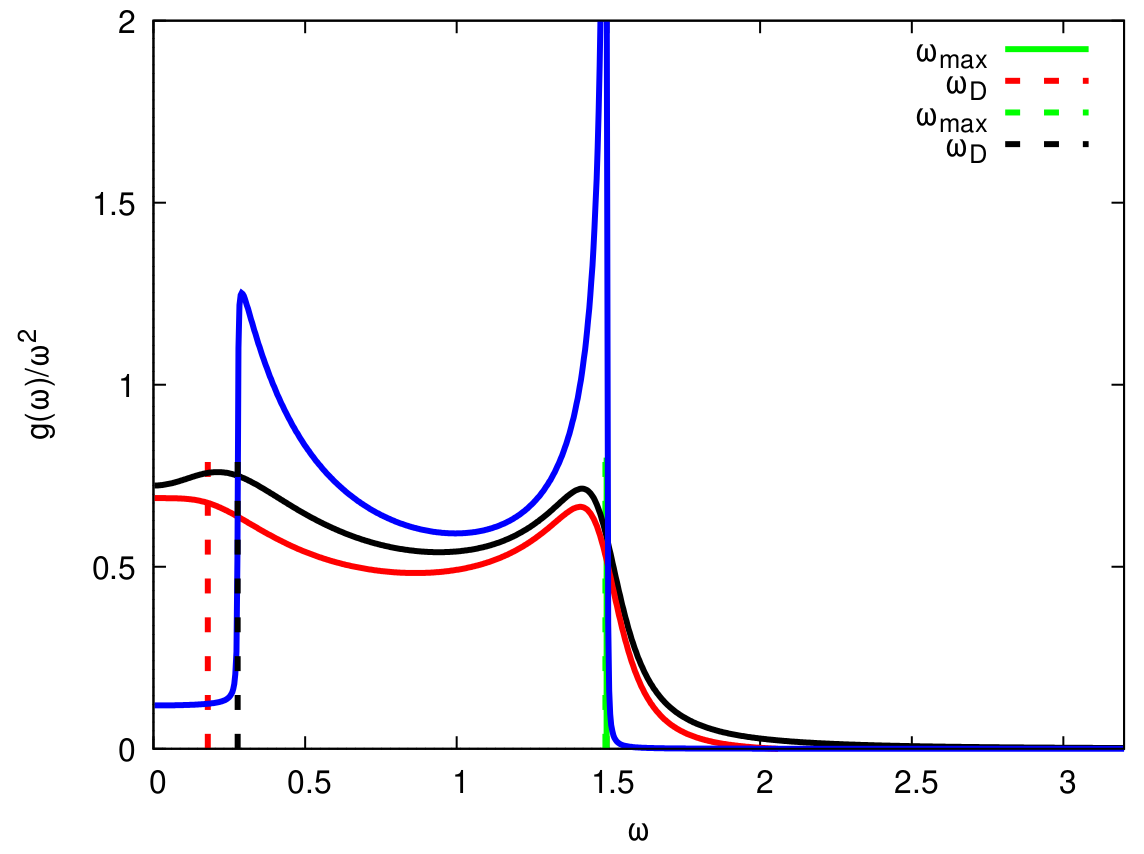Within the proton ordering model we explored the phenomenon of polarization rotation by external electric field in squaric acid, antiferroelectric crystals with parallel planes of hydrogen bonds. It has been shown that for the electric field, applied arbitrarily in these planes, the typical for antiferroelectrics reorientation of the sublattice polarization and associated transition to ferroelectric ordering, in the squaric acid is a two-step process. First, at a certain value of the field EII, one of the sublattice polarizations rotates by 90°, signaling the first order phase transition to the noncollinear ferrielectric phase with perpendicular sublattice polarizations (NC90), and only then, at higher fields (EIII), after the second polarization rotation by 90° the transition to the ferroelectric phase (FE) takes place. The low-temperature φ-E phase diagram is constructed (φ is the orientation angle of the external field); it is shown that EII ~1/cos(Δφ+π/4) and EIII~1/sin(Δφ+π/4), where φ is the angle between the electric field and initial polarization axis in the antiferroelectric phase. The constructed Т-E phase diagrams for different φ, apart from the mentioned antiferroelectric, noncollinear, and ferroelectric phases, contain the regions with a continuous polarization rotation, possible due to thermal fluctuations, as well as tricritical, and critical and double critісal end points (A.P. Moina, Phys. Rev. B, 103, 214104 (2021), https://doi.org/10.1103/PhysRevB.103.214104).The T-E1, phase diagram overlapping the color contour plot of θ, the vector between the sublattice polarizations. The phases, first and second order transition lines, various critical points, and experimental data are indicated.

In an effort to illuminate the general principles governing the critical behavior of model fluids we investigated how the shape and the (attractive) interaction range of the molecule affect the gas-liquid equilibrium and the critical behavior of the system. A combination of Monte Carlo simulations and analytical theory are employed to compute critical properties, i.e., temperature and density, of a system of hard-core ellipses with an attractive square-well potential in two-dimensional space. The critical temperature is found to decrease monotonically as the asphericity of the molecule is increased. This trend can be successfully explained in terms of the strength of the effective attraction acting between molecules measured, for instance, by the second virial coefficient. The critical density shows a complex dependence on both the range of attraction as well as on the asphericity of the molecule. We find that the properties of particle clusters formed in near critical states reproduce some of the most important features of the critical density, including multiple minima and maxima. It is shown that a model based on the extent of the overlap between attractive shells surrounding the ellipses captures the variation of the size of the clusters. Based on the obtained results we discuss implications of varying the shape of the attraction potential for critical density (R. Melnyk, Y. Kalyuzhnyi, G. Kahl, A. Baumketner, J. Chem. Phys., 2021, accepted).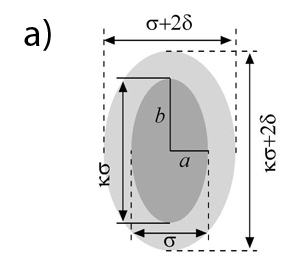a) Model of impenetrable ellipses with attractive layer. Particles interact only when their outer shells – shown as light gray, overlap. b) Critical temperature as a function of particle asphericity ratio κ and the thickness of the attraction shell δ с) Critical density as a function of the same variables as in b). Data obtained in computer simulations are shown along with the results of theoretical treatments.

Combining complex theoretical symmetry analysis, first principles lattice dynamics calculation and experimental Raman scattering measurements of SiO2+KH2PO4 nanocomposite, it is shown that the nanosized KH2PO4 crystals embedded in tubular silica pores of ~10-12 nm diameter preserve the main structural features of bulk monolithic single KH2PO4 crystals. The nano-scale confinement effect has a marginal impact on the KH2PO4 crystal structure. We observed some Raman intensity line redistribution in the nanocomposite sample, especially in its low-frequency range. Raman spectrum of KH2PO4+SiO2 nanocomposite (see Figure (b)) resembles in main features the spectrum taken from bulk KH2PO4 single crystal (see Figure (c)) (Ya. Shchur, A.V. Kityk, V.V. Strelchuk, A.S. Nikolenko, N.A. Andrushchak, P. Huber, A.S. Andrushchak, J. Alloys Compd., 868, 159177 (2021), https://doi.org/10.1016/j.jallcom.2021.159177).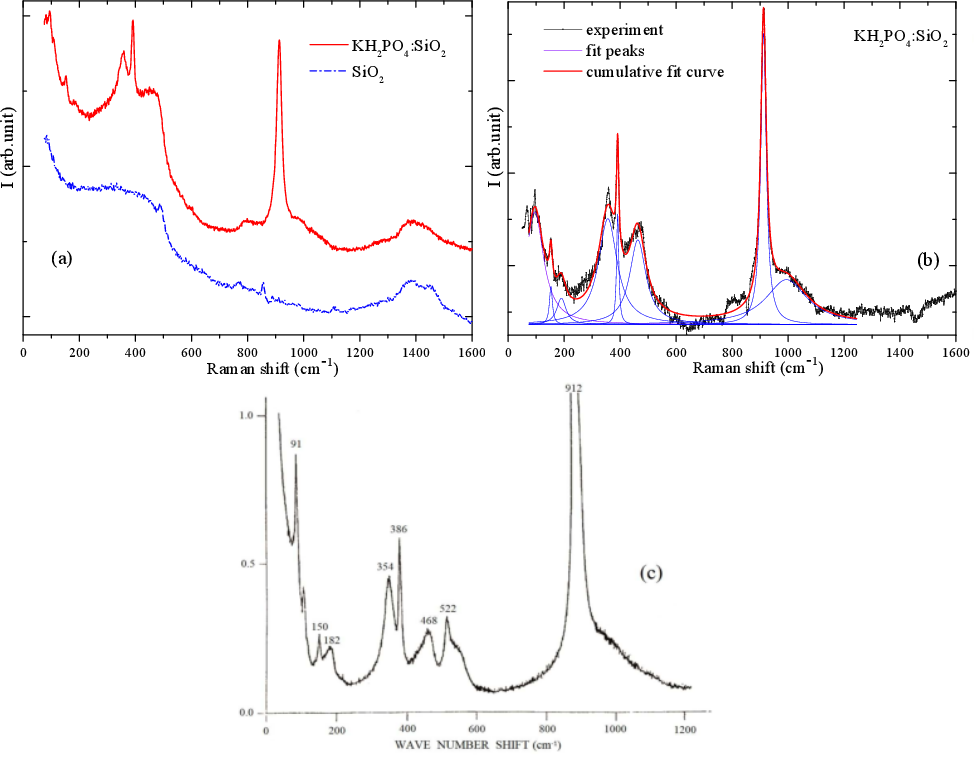The equilibrium properties of an adsorbate formed in the course of reactions A + B → ⊘ on a substrate with catalytic elements distributed inhomogeneously have been investigated. The two-dimensional substrate is modeled by the Bethe lattice [deep inside a loopless tree, figure (a)] with randomly distributed catalytic bonds with concentration p. For the symmetric case with equal chemical potentials of both species (μA = μB = μ) and equal interaction of the same species (JA = JB = J), the phase diagram was exactly calculated [figure (b)]. The existence of several phases is shown depending on J, μ, and p: a phase with a spontaneously broken symmetry between different species, in which they are present with different mean densities; a symmetric phase in which particles are present at the same densities, and the transitions between the phases can of both first-order and second-order. There are also two additional phases with structural ordering as a consequence of the lattice division into two sub-lattices: one of them is a phase in which both species appear predominantly on the same sub-lattice, leaving the second one almost empty, while in the second phase particles of the one type occupy predominantly one sub-lattice, while particles of another type appear mostly on the second one (D. Shapoval, M. Dudka, O. Bénichou, G. Oshanin, J. Phys. A: Math. Theor., 54, 385003 (2021), https://doi.org/10.1088/1751-8121/ac1c39].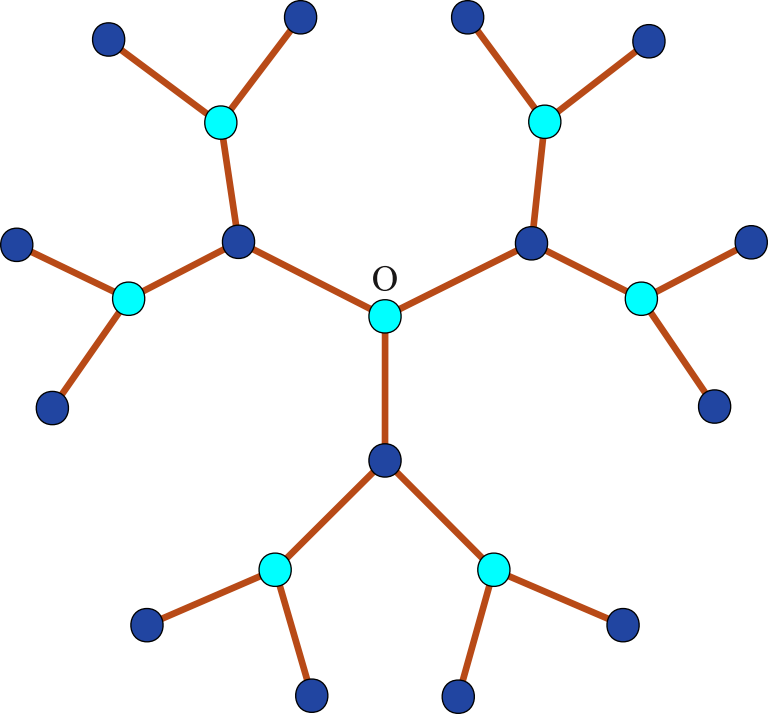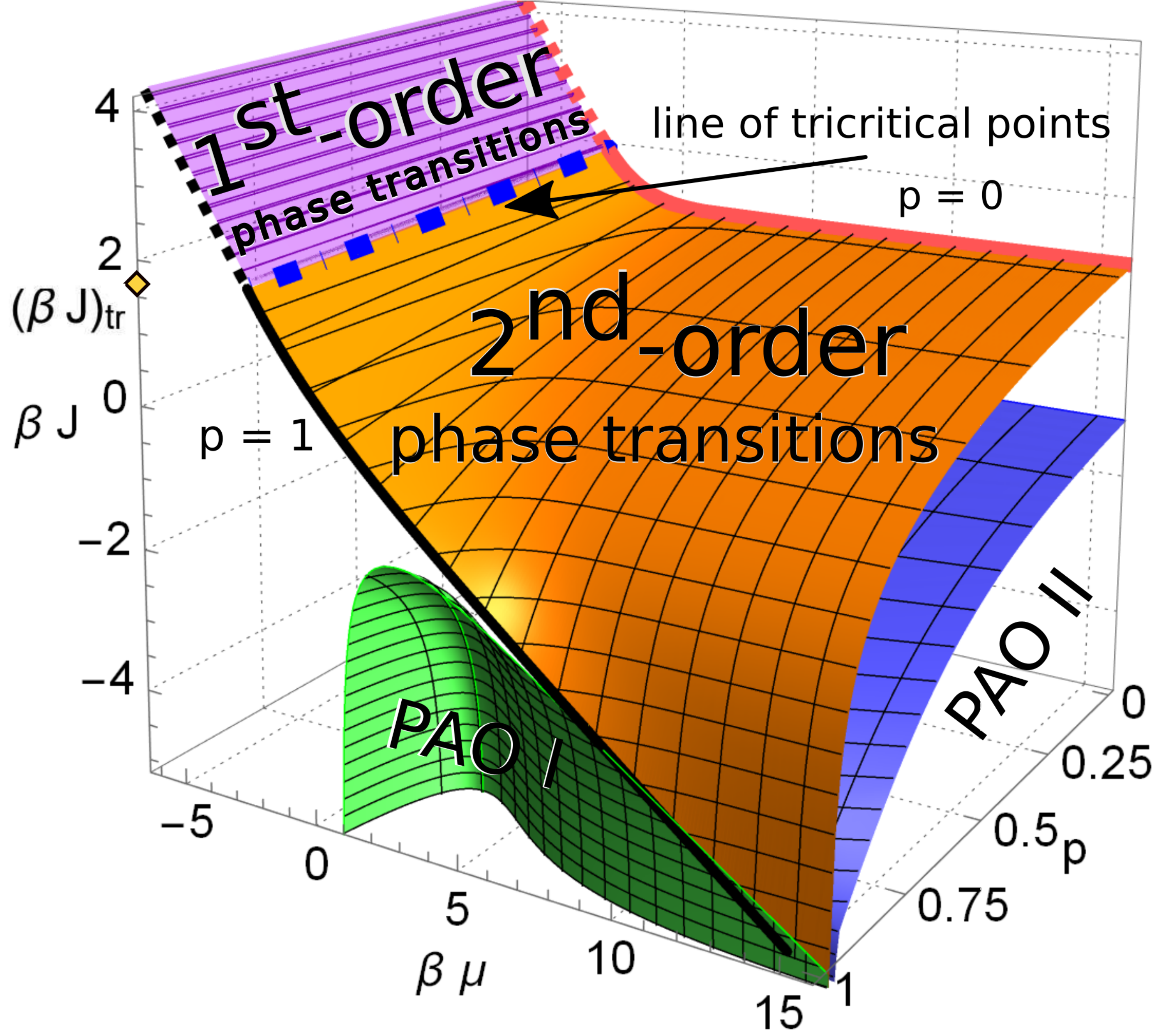The Bethe lattice with the coordination number q = 3 (a) and corresponding phase diagram of the two-component adsorbate (b), consisting of several phases: a phase with a broken symmetry (above the surface), a symmetric phase (below the surface), and phases with an alternating order (PAO I and PAO II).

The classical electrodynamics in flat spacetime of six dimensions has been studied. It describes a charged particle with rigidity, which is the first approximation of the Nambu-Goto string. The mass-shell relation of a free charge allows nonlinear oscillations parameterized by Jacobi elliptic functions. The motion of a charge in an external electromagnetic field is described by a nonlinear equation with higher derivatives. Having analyzed the problem of eigenvalues and eigenvectors of Faraday tensor, we established the algebraic structure of electromagnetic field in 6D. The classification scheme based on three field's invariants is thoroughly elaborated. Using the basic algebraic properties of the electromagnetic field tensor we examine the motion of a charge in a constant electromagnetic field. Its world line is a combination of hyperbolic and circular orbits that lie in three mutually orthogonal sheets of two dimensions. Within the braneworld scenario, we project the theory on the de Sitter space of four dimensions. As it turns out, spins of elementary particles themselves are manifestations of extra dimensions (Yu. Yaremko, J. Math. Phys., 62, No. 3, 032901 (2021), https://doi.org/10.1063/5.0023477).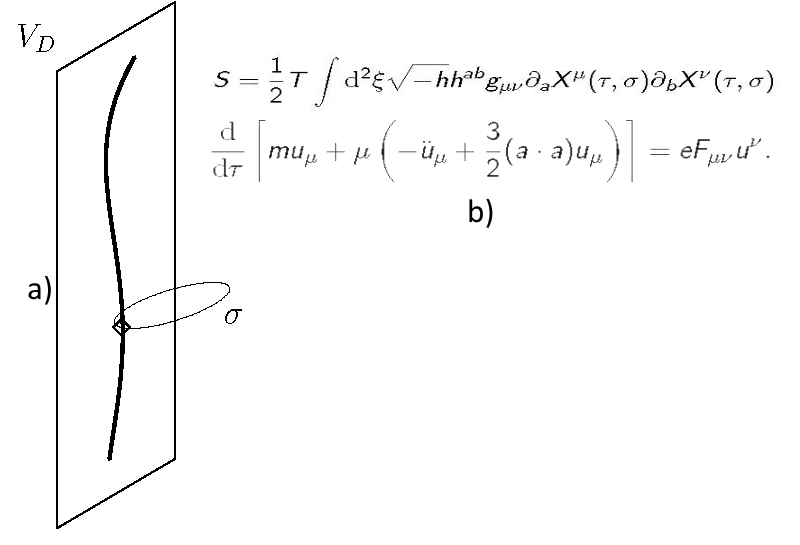(a) Schematic representation of charged particle as a string. (b) Renormalized classical electrodynamics in 6D, that describes charged particle with rigidity, which is the first approximation of the Nambu-Goto string (T is the string tension, gμν is the manifold metrics, hab is the ribbon metrics, ξ=(τ,ϭ)) and the non-linear equation of motion for the charge in electromagnetic field.

General physical background of famous Peres–Horodecki positive partial transpose (PH- or PPT-) separability criterion is revealed. Especially, the physical sense of partial transpose operation is shown to be equivalent to what one could call as the “local causality reversal” (LCR-) procedure for all separable quantum systems or to the uncertainty in a global time arrow direction in all entangled cases. Using these universal causal considerations brand new general relations for the heuristic causal separability criterion have been proposed for arbitrary DN × DN density matrices which describe the ensembles of N quantum systems of D eigenstates each. Resulting general formulas have been then analyzed for the widest special type of one-parametric density matrices of arbitrary dimensionality, which model a number of equivalent quantum subsystems being equally connected (EC-) with each other to arbitrary degree by means of a single entanglement parameter p. In particular, for the family of such EC-density matrices it has been found that there exists a number of N- and D-dependent separability (or entanglement) thresholds pth(N, D) for the values of the corresponded entanglement parameter p, which in the simplest case of a qubit-pair density matrix in H2 ⊗ H2 Hilbert space are shown to reduce to well-known results obtained earlier independently by Peres (Phys. Rev. Lett., 77, 1413 (1996)) and Horodecki (Phys. Lett. A, 223, 1–8 (1996)). As the result, a number of remarkable features of the entanglement thresholds for EC-density matrices has been described for the first time. All novel results being obtained for the family of arbitrary EC-density matrices are shown to be applicable to a wide range of both interacting and non-interacting (at the moment of measurement) multi-partite quantum systems, such as arrays of qubits, spin chains, ensembles of quantum oscillators, strongly correlated quantum many-body systems with the possibility of many-body localization, etc. (G. Skorobagatko, Sci. Rep., 11, 15866 (2021), https://doi.org/10.1038/s41598-021-94804-2).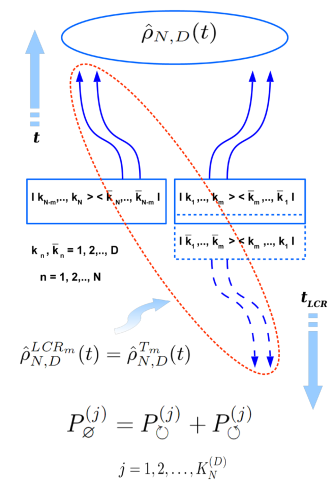Schematic picture showing a possibility of local causality reversal procedure for all separable quantum density matrices and the impossibility of such a procedure for all those density matrices encoding the entangled quantum states of entire quantum system (ensemble). In the former case the fundamental uncertainty with respect to the direction of a local causal evolution for separable subsystem takes place, while in the latter case the fundamental uncertainty with respect to a global time arrow direction is evident for the entangled subsystems of the entire quantum system (ensemble).

The study aims for enhancing our understanding of the peculiarities related to the formation of multi-enzyme complexes, which can increase the efficiency of cellulose hydrolysis in the biofuel production. By combining computer simulation, theory and experiment, we focus on developing optimal structures of polymer scaffolds for synthetic cellulosomes and on finding optimal scenarios for their practical application. We have developed the coarse-grained model of enzyme-polymer complexes with different molecular architectures and, using the method of computer simulations, investigated the process of their self-assembly in mixtures of polymer scaffolds and free enzymes. Since the synergy of biological complexes arises due to the strict order of the enzymes positions on scaffolds, the obtained model complexes are analyzed in terms of the specific sequences (motifs) of enzymes. It is found that enzyme-polymer complexes adsorbed on the cellulose surface lead to a significant increase in the population of certain motifs when compared to the case of adsorption of a mixture of free enzymes. The effect can be enhanced by increasing the length of a given sequence and becomes sufficiently strong at small adsorption density, what is important from the point view of efficiency of the complexes for hydrolysis (O. Zholobko, A. Hammed, A. Zakharchenko, N. Borodinov, I. Luzinov, B. Urbanowicz, T. Patsahan, J. Ilnytskyi, S. Minko, S.W. Pryor, A. Voronov, ACS Appl. Polym. Mater., 3, 1840 (2021), https://doi.org/10.1021/acsapm.0c01407).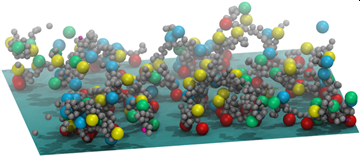Concepts in a certain domain of science are linked via intrinsic connections reflecting the structure of knowledge. To get a qualitative insight and a quantitative description of this structure, we perform empirical analysis and modeling of the network of scientific concepts in the domain of physics. To this end we use a collection of manuscripts submitted to the e-print repository arXiv and the vocabulary of scientific concepts collected via the ScienceWISE.info platform and construct a network of scientific concepts based on their co-occurrences in publications. The resulting complex network possesses a number of specific features (high density, dissortativity, structural correlations, skewed node degree distribution) that cannot be understood as a result of simple growth by several commonly used network models. We show that the model based on a simultaneous account of two factors, growth by blocks and preferential selection, gives an explanation of empirically observed properties of the concepts network (V. Palchykov, M. Krasnytska, O. Mryglod, Yu. Holovatch, Condens. Matter Phys., 24, No. 2, 24001 (2021), https://doi.org/10.5488/CMP.24.24001; Adv. Complex Syst., 24, 2140001 (2021), https://doi.org/10.1142/S0219525921400014).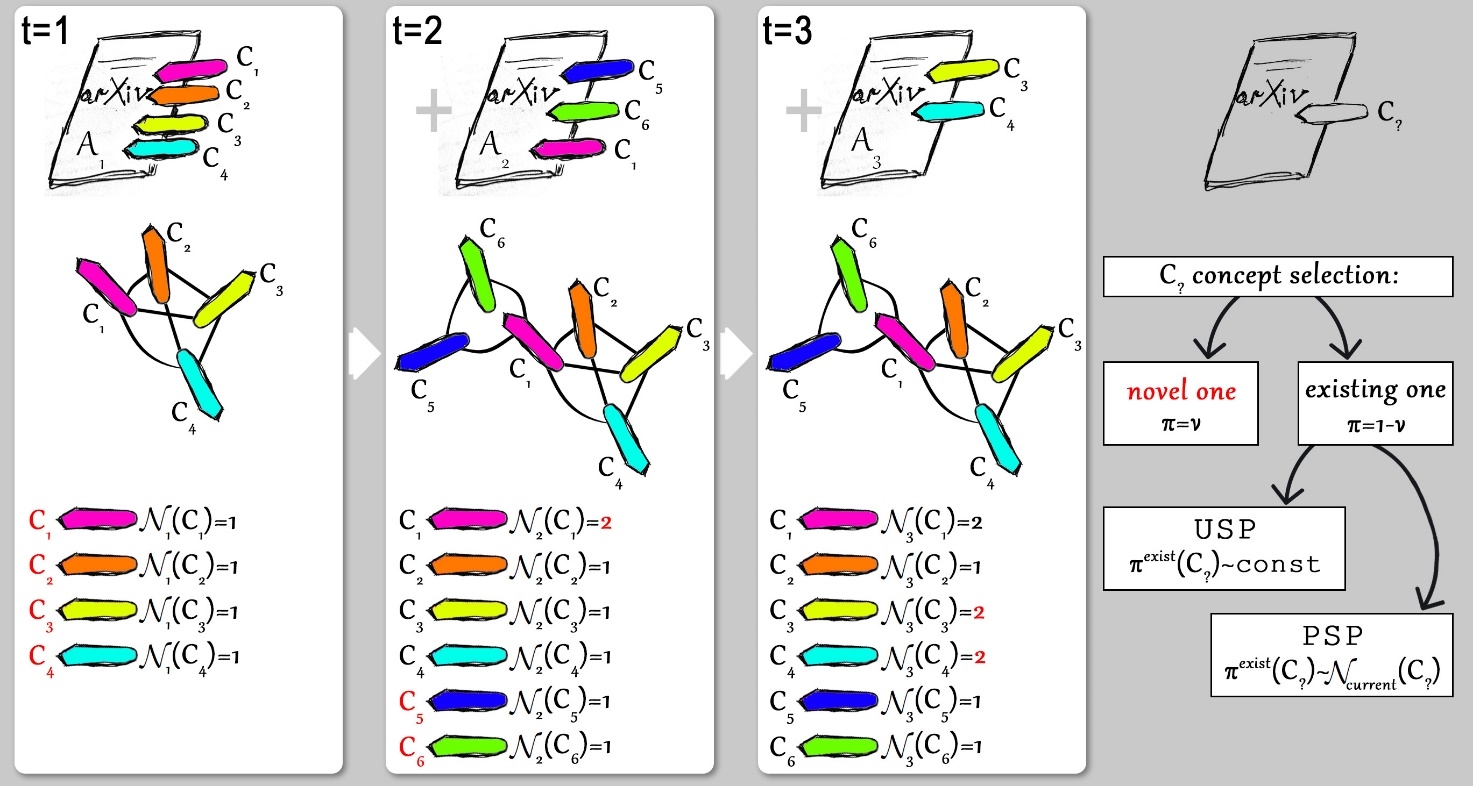Model demonstration. At each time step t a new article is generated. An article At containing nt concepts enters the concepts network as a complete graph, and affects the number of occurrences Nt(Ct) of a concept Ci. Once a new article At is being generated, all nt concepts slots should be filled out in a probabilistic way. A concept Ci may be either a novel one (the one introduced in the article At for the first time), or one the concepts that appeared on previous time steps. The model considers two scenarios for existing concept selection: uniform selection process (USP) and preferential selection process (PSP).